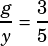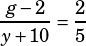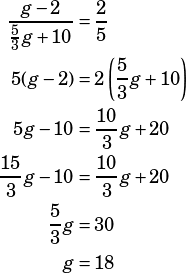A bag contains mangoes that are either green or yellow. The ratio of green mangoes to yellow mangoes in the bag is 3 to 5. When two green mangoes are removed and ten yellow mangoes are added, the ratio becomes 2 to 5. How many green mangoes were originally in the bag?

Say that the original number of green mangoes is g and the original number of yellow mangoes is y. So you know that.

When two green mangoes are removed, and 10 yellow mangoes are added, the ratio becomes.

Now we have two equations and two variables—we can solve for g to get the answer we seek!

Manipulate the first equation to set it equal to y:Now substitute into the other equation:You can check your work on this easily enough. If there were originally 18 green mangoes and the ratio of green to yellow was 3 to 5, then there were originally 30 yellow mangoes. What happens if you take away 2 green mangoes and add 10 yellow mangoes? You end up with 16 green and 40 yellow, which simplifies to a ratio of 2 to 5, just like the question said.

One last note here. You didn’t provide answer choices for this question so I assume there weren’t any. However, if there were answer choices here, this would be an ideal question to backsolve—essentially to “check your work” like we just did in the last paragraph without doing the work!